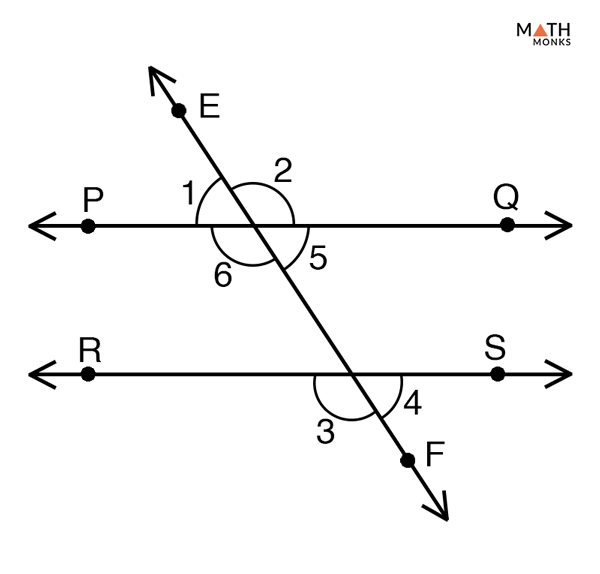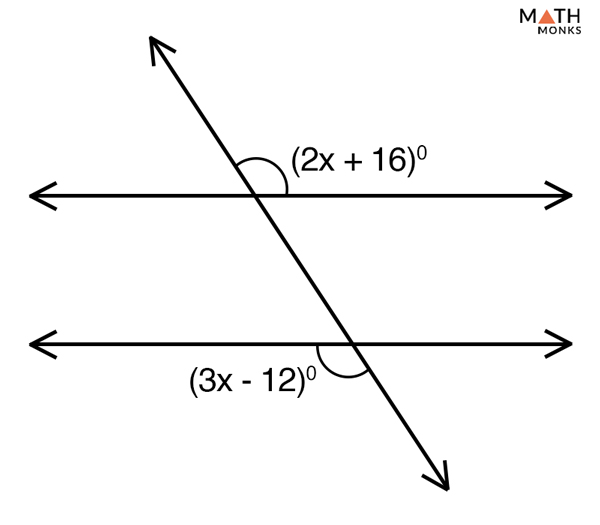# Alternate Exterior Angles

## What are Alternate Exterior Angles

Alternate exterior angles are the pairs of angles that are formed when a transversal intersects two parallel or non-parallel lines. They are found on the outer side of two parallel lines but on opposite side of the transversal.

When the two lines are parallel the alternate exterior angles are found to be equal.

The above figure shows two parallel lines AB and CD intersected by the transversal PQ. The two pairs of alternate exterior angles formed on the opposite side of the transversal but outside of two parallel lines are identified as:

• ∠1 and 2
• ∠3 and ∠4

Thus ∠1 = ∠2 and ∠3 = ∠4

## Properties

1. They are congruent if the two lines crossed by the transversal are parallel
2. If the alternate exterior angles are congruent then the two lines are parallel
3. They are supplementary if the transversal intersects two parallel lines at right angles.

## Alternate Exterior Angles TheoremProve Alternate Exterior Angles Theorem

To prove:

∠1 = ∠4 and ∠2 = ∠3

Proof:

Let PQ and RS be the two parallel lines intersected by the transversal EF
To start with we will denote the vertically opposite angle of ∠1 as ∠5 and that of ∠2 as ∠6
Thus,
∠1 = ∠5 (Vertically Opposite Angles)
Now,
∠5 = ∠4 (Corresponding Angles)
∠1 = ∠4 (Transitive Property of Congruence)
Similarly,
∠2 = ∠3
Hence Proved

### The converse of Alternate Exterior Angles TheoremProve the Converse of Alternate Exterior Angles Theorem

To prove:

PQ∥ RS

Proof:

Let ∠1 = ∠4 and ∠2 = ∠3 be the two pairs of congruent alternate exterior angles.
Thus,
PQ∥ RS (by Alternate Exterior Angles Theorem)
Hence Proved

Let us take some examples to understand the concept better.Find the value of p and q in the given figure.

Solution:

As, ∠66° = ∠q (Alternate exterior angles)
Hence, ∠q =66°
Similarly,
∠114° = ∠p (Alternate exterior angles)
Hence, ∠p =114°Solve the value of x in the given figure.

Solution:

As we know, the alternate exterior angles are congruent
Thus,
(2x + 16)° = (3x – 12)°
3x -2x = 16° + 12°
x = 28°

Two alternate exterior angles are (2x – 14)° and (x + 4)° Prove whether the two exterior angles are congruent.

Solution:

According to the exterior angle theorem, alternate exterior angles are equal when the transversal crosses two parallel lines.
Thus,
(2x – 14)° = (x + 4)°
2x –x = 14 + 4
x = 18°
Now, substituting the value of x in both the exterior angles expression we get,
(2x – 14)° = 2 x 18 – 14 = 22°
(x + 4)°= 18° + 4 = 22°
Hence, the two exterior angles are congruent, which further proves that the two lines that are intersected by the transversal are parallel.

## Examples in Real Life

• In buildings, bridges, roads, and railings
• In fitting items such as sofas, chairs, and tables
• In set-squares
• Daily items such as scissors, partly opened-doors, cycle spokes, arrowhead, and pyramids